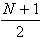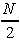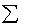previous lesson

Central Tendency

NOMINAL DATA: Summarizing Univariate Distributions

A. Central tendency: mode = the category with the largest frequency

Example: Rape: Relationship of offender to the victim, Kansas, 1988

 RELATIONSHIP f ACQUAINTANCE 219 STRANGER 213 FRIEND 47 HUSBAND/COMMON-LAW HUSBAND 32 EX-BOYFRIEND 31 BOYFRIEND 10 EX-HUSBAND 15 FATHER/STEP FATHER 20 BROTHER 8 GRANDFATHER 2 UNCLE 1 OTHER FAMILY/IN-LAW 14 UNKNOWN 141 TOTAL 782

SOURCE: Caputi, J. & D. E. H. Russell "Femicide" Speaking the Unspeakable" MS Sept./Oct., 1990

Q: What is the average or typical relationship of a rapist to the victim?

A: Look at variable and decide what type it is: This is nominal data. Look at the question. It is asking for central tendency. Mode = acquaintance

*If two categories are both equally large, there will be 2 modes. There will be no central tendency if all categories have the same frequency.

ORDINAL DATA: Summarizing Univariate Distributions

A. Median: Central tendency

1. Put variable in order.

2. Number of median is:

a) If N is an odd number,1. If N is an even number, the median(s)  is/are bothandif they are different ordinal values.  For example, if the set of ordinal values is 3,3,6,7,8,8; then the median would be both 6 and 7 as there is no ordinal value 6.5 in the set.

Example: Nudity Tolerance Score: (ungrouped data)

 La Jolla San Diego 91 98 90 98 87 83 86 83 N = 12 85 83 N = 11 median -> 83 82 <- median median -> 81 82 78 71 77 42 76 31 76 30 73

Q: What is the average score in La Jolla? In San Diego?

A: In La Jolla, the median is both 83 and 81. In San Diego, the median is 82.

Example: Past Soc201 final grades (grouped data, ordinal w/odd number of cases)

 Grade f Cum f Cases A 16 16 1-16 B 18 34 17-34 C 21 55 35-55 D 23 78 56-78 F 13 91 79-91 N = 91

Q: What is the average grade for Soc201 classes?

A: The average grade for Soc201 is "C." Since N is odd,

(N + 1) / 2 = (91 + 1) / 2 = 46. The median is the ordinal category that contains case # 46 not 46!

Example: Persons who have known someone with AIDS (grouped data, ordinal w/even number of cases).

 SES (socio-economic status) f cum f cases low 190 190 1-190 middle 221 411 191-411 high 33 444 412-444 N = 444

SOURCE: GSS73-91 SURVEY SUBSET

Q: What is average SES of persons who have known someone with AIDS?

A: Median SES "middle class"

444 / 2 = 222, 444+2 / 2 =223 the median falls between cases 222 & 223.

Interval Data or continuous Ordinal Data: Summarizing Univariate Distributions

A. Median: Central tendency

In some instances, such as income, housing costs, etc., a small minority of people skew the mean average (i.e. inflate or deflate). Additionally, some ordinal data is rendered in a numerical form (i.e. IQ scores, GPA's, etc.). In these cases, the median average is used. The following formula allows for a precise numerical answer. This formula is ordinarily used for grouped data with intervals greater than 1.

Median (Mdn)= LTL + {i[N(.50) - cfbelow ]/ f }

where cfbelow = cumulative frequency below interval containing the median.

f = the number of cases in the interval containing the median.

i = width of interval

N = total number in sample

LTL = lower true limit of the interval containing the median

Example: Ages (at last birthday)

 Md Ages Cum f 18-19 19.00 23 23 20-21 21.00 44 67 22-23 23.00 44 111 24-25 25.00 52 163 26-27 27.00 50 213 28-29 29.00 79 292 30-31 31.00 74 366 32-33 33.00 66 432 34-35 35.00 72 504 36-37 37.00 83 587 38-39 39.00 85 672 40-41 41.00 79 751 42-43 43.00 72 823 44-45 45.00 62 885 885

• Find middle case. N(.50) = 885(.50) = 442.5
• Find interval that contains the middle case. cfbelow is the cumulative frequency of the category immediately before the median category. cf below = 432.
• Figure the proportion of the interval the median category is. [N(.50) - cfbelow] / f = (442.5 - 432) / 72 = .146
• Multiply by i (i = real upper limit - real lower limit of each interval - must be a consistent interval) 35.999 - 34.000 = 2. 2(.146) =.292
• Add to real lower limit of median interval - 34.000 + .292 = 34.29 years.
• Q: What is the median average age?
A: The median average age is 34.29 years.

B. Mean: Central tendency (abbreviatedcalled x-bar)

1. ungrouped data:= x / N

Where: x = variable

N = total in sample

Example:

 weight 80 N = 3 90 60 230 =x

Q: What is the average weight? There are 3 meanings of "average": nominal data = mode, ordinal data = median, interval data = mean (unless disproportionately skewed by upper or lower end of the range in which case median is used.)

A: = 230 / 3 = 76.67

2. grouped data:=fm / N

Where: f = frequency of a category

m = midpoint of a category

N = total number of cases in sample

 # of children f m fm 1 - 3 400 2 800 4 - 6 300 5 1500 7 - 9 200 8 1600 10 - 11 100 11 1100 1000 = N 5000 =fm

Example:

Q: What is the average number of children?

A: 5000 / 1000 = 5